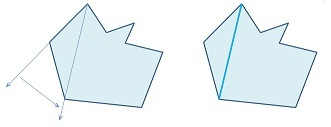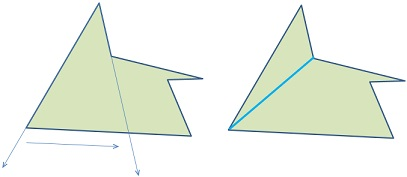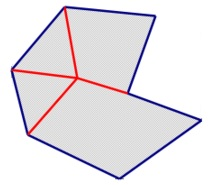## Jordan Curve Theorem, Proof# JCT - Abstract Graphs and Euler’s Formula

Scott E. Brodie, MD, PhD
Icahn School of Medicine at Mount Sinai
New York

• An Abstract Graph (or simply a graph) is a finite set of points (here called vertices), together with a list of (unordered) pairs of distinct vertices, (here called edges).

• The graph is planar or can be realized in the plane if there is an arrangement of points and arcs in the plane such that the arcs are disjoint, except for their extremities, and there exists an arc in the plane between vertices exactly when there is an unordered pair of the abstract graph comprising the two endpoints of the segment.

• A graph is 2-connected if every arc is part of a Jordan polygon comprising edges of the graph. A face of a planar graph is a component of the complement of the set of edges.

• As the realization of a graph in the plane is a compact set, its complement contains a unique unbounded component.

### Plane Geometry and Euler’s Formula for a plane graph

• As we have proved Jordan separation for polygons, we may now discuss the interior and exterior of any polygon.

• The interior angles of a triangle sum to $\pi.$

• The sum of all the angles at a point in the plane is $2\pi.$

• By drawing segments from an internal point to each vertex, one sees that the interior angles of a convex polygon of sides sums to $n\pi - 2\pi=(n-2)\pi.$• In fact the interior angles of any polygon sum to $(n-2)\pi\ldots$

• This follows from the fact that any polygon can be triangulated by diagonals (segments connecting nonadjacent vertices such that the segments lie entirely in the interior of the polygon).

• To verify this, we proceed by induction. The statement is easily seen to hold for triangles.

• For $n>3,$ orient the polygon so no two vertices lie on a horizontal line. From the top-most vertex, swing a ray from one adjacent edge to the other, choosing the direction such that the vertex angle is less than $\pi.$

• If this ray intersects another vertex before reaching the adjacent edge, then the topmost vertex can be connected to that vertex by a diagonal. Temporarily separate the polygon along this diagonal into two polygons with fewer than n vertices, and triangulate each sub-polygon by diagonals. Now recombine.• If the ray encounters no vertices before reaching the adjacent edge, then the two vertices adjacent to the opmost vertex can be connected by an interior diagonal, and we may again proceed as above.It is easily seen by induction that such a triangulation results in $n-2$ triangles, each of which contributes $\pi$ to the sum of interior angles, and we are done.

• Thus, the sum of the internal angles of a bounded face of a 2-connected graph is

$(V_f - 2)\pi = (E_f\;–\,2)\pi,$

where $V_f$ is the number of vertices (and thus also the number of edges, $E_f)$ of the face.

• But the sum of all the angles at all the vertices of a planar graph with $V$ vertices is $2\pi V.$

• So the sum of the external angles of a polygon with $n$ vertices (or $n$ sides) is therefore given by the sum of all the angles minus the sum of the internal angles:

$2\pi n - \pi (n-2) = (n+2)\pi.$

Now consider a 2-connected planar graph. We distinguish the edges which form part of the boundary of the unbounded face (the exterior edges) from those which do not (the interior edges).Then the sum of all the angles at all the vertices of a 2-connected graph is also given by ...

\displaystyle\begin{align} 2\pi V &= \sum(\text{all the internal angles of the bounded faces})\\ &+ \sum(\text{all the external angles of the bounding polygon})\\ &=\sum_{\text{over internal faces}}(E_f\;– 2)\pi +(E_e + 2)\pi\\ &= 2(\#\text{Edges})\pi - 2\pi (\#\text{Internal faces}) +2\pi, \end{align}

as each internal edge occurs twice in the sum over faces (see above), and the external edges once in the sum over faces, and once in the next term, and thus also appear twice as well.

• Dividing by $2\pi,$ we obtain

• $V = E – F + 1,\;$ or

• $F-E+V = 1,$

where $F$ is the number of internal faces.

• If we now agree to count the unbounded component of the complement of the 2-connected graph as a "face", we obtain Euler's formula:

• $F-E+V = 2.$

• For the case of a polygon, $E = V,$ so Euler's formula gives $F = 2,$ confirming JCT for polygons (!!)## Jordan Curve Theorem, Proof